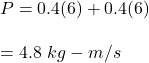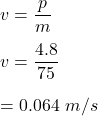## Two skaters, both of mass 75 kg, are on skates on a frictionless ice pond. One skater throws a 0.4-kg ball at 6 m/s to his friend, who catch

Question

Two skaters, both of mass 75 kg, are on skates on a frictionless ice pond. One skater throws a 0.4-kg ball at 6 m/s to his friend, who catches it and throws it back at 6.0 m/s. When the first skater has caught the returned ball, what is the velocity of each of the two skaters

in progress 0
6 months 2021-07-17T21:13:54+00:00 1 Answers 8 views 0

v = 0.064 m/s

Explanation:

Given that,

The mass of two skaters = 75 kg

The mass of a ball = 0.4 kg

The speed of the ball = 6 m/s

The speed of skater = 6 m/s

We need to find the velocity of each of the two skaters.

Under the values given the moment with respect to the ball and which is subsequently transmitted to people it would be given by:We know that,

P = mv

Where

v is the velocity of each skater.So, the velocity of each of the skaters is 0.064 m/s.